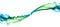Composing Software: An IntroductionSmoke Art Cubes to Smoke — MattysFlicks — (CC BY 2.0)

Composing Functions

```const g = n => n + 1;
const f = n => n * 2;const doStuff = x => {
const afterG = g(x);
const afterF = f(afterG);
return afterF;
};doStuff(20); // 42```
```const g = n => n + 1;
const f = n => n * 2;const wait = time => new Promise(
(resolve, reject) => setTimeout(
resolve,
time
)
);wait(300)
.then(() => 20)
.then(g)
.then(f)
.then(value => console.log(value)) // 42
;```
```const g = n => n + 1;
const f = n => n * 2;const doStuffBetter = x => f(g(x));doStuffBetter(20); // 42```
```const doStuff = x => {
const afterG = g(x);
console.log(`after g: \${ afterG }`);
const afterF = f(afterG);
console.log(`after f: \${ afterF }`);
return afterF;
};doStuff(20); // =>
/*
"after g: 21"
"after f: 42"
*/```
```const trace = label => value => {
console.log(`\${ label }: \${ value }`);
return value;
};```
```const doStuff = x => {
const afterG = g(x);
trace('after g')(afterG);
const afterF = f(afterG);
trace('after f')(afterF);
return afterF;
};doStuff(20); // =>
/*
"after g: 21"
"after f: 42"
*/```
```import pipe from 'lodash/fp/flow';const doStuffBetter = pipe(
g,
trace('after g'),
f,
trace('after f')
);doStuffBetter(20); // =>
/*
"after g: 21"
"after f: 42"
*/```
```// pipe(...fns: [...Function]) => x => y
const pipe = (...fns) => x => fns.reduce((y, f) => f(y), x);```

Composing Objects

```const firstName = 'Claude';
const lastName = 'Debussy';```
```const fullName = {
firstName,
lastName
};```
```class Foo {
constructor () {
this.a = 'a'
}
}class Bar extends Foo {
constructor (options) {
super(options);
this.b = 'b'
}
}const myBar = new Bar(); // {a: 'a', b: 'b'}```
```const a = {
a: 'a'
};const b = {
b: 'b'
};const c = {...a, ...b}; // {a: 'a', b: 'b'}```